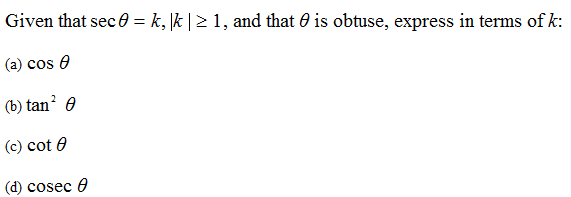# Simple trig questionI'm just curious on how to find out what quadrant θ is in from the information. I know it says that theta is obtuse, but doesn't this only conclude that theta is not in the first quadrant? In the solutions they have right away said theta is obtuse therefore it is in the second quadrant so k is < 0, which I can understand but couldn't theta be in the 3rd & 4th quadrant also? Also what is |k|≥ 1 used for?

EDIT: nevermind, I though obtuse was just >90, not between 90 and 180 :x - If anyone could answer what |k| is for it'd help thanks.

Mentallic
Homework Helper
The name for angles that are between 180 and 360 are called reflex.

And as for the $|k|\geq 1$ this is probably just enforcing that $\theta$ is real.

The name for angles that are between 180 and 360 are called reflex.

And as for the $|k|\geq 1$ this is probably just enforcing that $\theta$ is real.

thanks again.